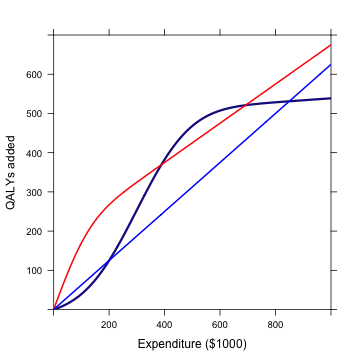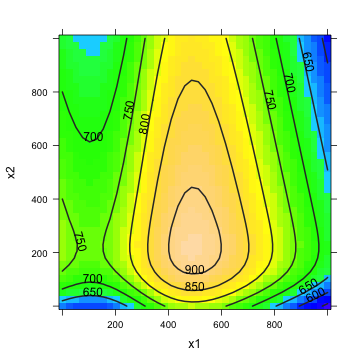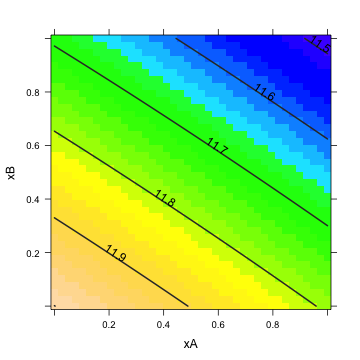# QALY Problem 2

In a previous exercise, a graph of the hypothetical impact of three forms of health-care expenditures was presented. Here is a similar exercise, which will explore a constrained optimization using slightly hypothetical functions, $$f_1$$, $$f_2$$, and $$f_3$$:

f1 = makeFun(500 * pnorm(x, mean = 300, sd = 150) + 0.05 * x - 11.375 ~
x)
f2 = makeFun(350 * pnorm(x, mean = 0, sd = 100) + 0.5 * x - 175 ~
x)
f3 = makeFun(x/1.6 ~ x)The problem is how to allocate the available funds, $1,000K, among the different health-care interventions. One strategy for solving constrained optimization functions is to use the constraint to reduce the “dimension'' of the problem. In the unconstrained problem, there are three quantities to be set: $$x_1$$, $$x_2$$, and $$x_3$$, the amount of money spent on each intervention. The constraint imposes a relationship among the three quantities, namely that $$x_1 + x_2 + x_3 = 1000$$. This means that there are really only two independent quantities, since setting two of the quantities determines the value of the third: a reduction in dimension from three to two. To see this, construct the overall output from any pattern of expenditure meeting the constraint and plot it out: plotFun(f1(x1) + f2(x2) + f3(1000 - x1 - x2) ~ x1 & x2, x1.lim = range(0, 1000), x2.lim = range(0, 1000))Make sure that you understand why the overall function has been constructed this way: $$x_1$$ is the amount spent on intervention one, $$x_2$$ is the amount spent on intervention two, leaving $$1000-x_1-x_2$$ to be spent on intervention three. The constraint, rather than being graphed, has been used to turn a function of three variables $$x_1$$, $$x_2$$, and $$x_3$$, into a function of just two variables. From the graph you can read off the values of $$x_1$$ and $$x_2$$ that maximize the overall function subject to the constraint. Then you can use the constraint to find the value of $$x_3$$. To get a more precise estimate of the location of the maximum, remake the plot in a narrow region around the peak. What are the values of $$x_1$$ and $$x_2$$ at the optimum? • $$x_1$$: \SelectSetHoriz{494.25}{475.25,483.25,494.25,498.25} • $$x_2$$: \SelectSetHoriz{219.70}{219.70,221.70,223.70,225.70} The derivative of the functions tells how much the output changes for an additional dollar of expenditure. Calculate the derivatives at the optimum point: • For function 1: $$\frac{d f_1}{d x_1}$$ \SelectSetHoriz{0.625}{0.000,0.500,0.523,0.625,1.20,1.37} • For function 2: $$\frac{d f_2}{d x_2}$$ \SelectSetHoriz{0.625}{0.000,0.500,0.523,0.625,1.20,1.37} • For function 3:$$\frac{d f_3}{d x_3}$$ \SelectSetHoriz{0.625}{0.000,0.500,0.523,0.625,1.20,1.37} \begin{AnswerText} r df1 = D(f1(x) ~ x) df2 = D(f2(x) ~ x) df3 = D(f3(x) ~ x) df1(x = 494.25)   ##  0.05  r df2(x = 219.7)   ##  0.5  r df3(x = 1000 - 494.25 - 219.7)   ##  0.625  \end{AnswerText}  Explain what aspect of your answer to the above questions about the derivative tells you that your values of $$x_1$$, $$x_2$$ and $$x_3$$ are optimum? \TextEntry \begin{AnswerText} All the derivatives are the same at the optimal point. This reflects the optimum in that any small reallocation of funds from one intervention to the next will result in no net change in output. If the derivatives of the different interventions were not the same, it would be possible to improve the overall outcome by a reallocation. \end{AnswerText}  ### Toward a More Realistic Setting The optimization techniques described above are completely realistic, but it's not so realistic to have specific formulas for the relationship between expenditures and outcomes. Somewhat more realistically, you might have the opinions of experts about the outcomes, in the form of a table like this. \begin{centering} \begin{tabular}{lrrrrrr} Expenditure & 0 & 10 & 20 & 30 & 40 & 50\\\hline A & 0 & 4 & 10 & 15 & 18 & 24\\ B & 0 & 3 & 8 & 19 & 25 & 30\\ C & 0 & 6 & 12 & 18 & 26 & 31\\ \end{tabular} \end{centering}  Such estimates from experts should be taken with a grain of salt, but they are often the best information you have to inform a model. You can turn the expert's opinions into functions by using splines. In this case, there is good reason to think that output will increase monotonically with expenditure, so a monotonic spline is a good choice. You can construct the functions like this: expend = c(0, 10, 20, 30, 40, 50) A = c(0, 4, 10, 15, 18, 24) B = c(0, 3, 8, 19, 25, 30) C = c(0, 6, 12, 18, 26, 31) dat = data.frame(expend = expend, A = A, B = B, C = C) fA = spliner(A ~ expend, data = dat, monotonic = TRUE) fB = spliner(B ~ expend, data = dat, monotonic = TRUE) fC = spliner(C ~ expend, data = dat, monotonic = TRUE)  Find the best values of inputs $$x_A$$, $$x_B$$, and $$x_C$$ given the constraint that total expenditure is $$x_A + x_B + x_C = 50$$. Choose the closest answer: • Input to A: $$x_A$$ \SelectSetHoriz{0}{0,5,10,15,21,24,30,34,39,43,50} • Input to B: $$x_B$$ \SelectSetHoriz{34}{6,11,16,19,25,29,34,41,43,50} • Input to C: $$x_C$$ \SelectSetHoriz{16}{7,11,16,19,21,25,29,34,39,41,44} \begin{AnswerText} r overall = makeFun(fA(xA) + fB(xB) + fC(50 - xA - xB) ~ xA & xB) plotFun(overall(xA = xA, xB = xB) ~ xA + xB, xA.lim = range(0, 50), xB.lim = range(0, 50))  ![plot of chunk splineans](figure/splineans.png) The optimum is near $$x_A=0$$, $$x_B=34$$ and therefore $$x_C = 50 - 34 - 0 = 16$$. This gives an output of about 31.4 units. r overall(xA = 0, xB = 34)   ##  31.41  \end{AnswerText}  The American Association of Allergy Activists (AAAA) has lobbied Congress to mandate that, of the 50 units of available funds, funding for A must be at least $$x_A \geq 30$$ with only the remaining 20 units of expenditure available to be allocated to B and C. How much would this constraint reduce the overall output for the three interventions combined? (Remember, if you're spending 30 on A, you can't spend more than 20 on B.) \begin{MultipleChoice} \wrong{Not at all.} \wrong{About zero to 1 output unit.} \correct{About 4 to 5 output units.} \wrong{About 10 to 12 output units.} \wrong{About 14 to 16 output units.} \wrong{It would actually {\bf increase} the output.} \end{MultipleChoice}  \begin{AnswerText} Looking at the contour plot along the path $$x_A = 30$$ indicates that the best possible outcome will be about 28 units. This is a reduction of about 3 units from the optimum when $$x_A$$ is not subject to the proposed Congressional constraint. \end{AnswerText}  Good news and bad news. You've defeated the AAAA initiative to force expenditure on A. But, regretably, general budget cuts have just been announced! Now there are only 20 units to spend on the three interventions. What's the best mixture? \begin{itemize} \item Input to A: $$x_A$$ \SelectSetHoriz{0}{0,5,10,15,20} \item Input to B: $$x_B$$ \SelectSetHoriz{0}{0,5,10,15,20} \item Input to C: $$x_C$$ \SelectSetHoriz{20}{0,5,10,15,20} \end{itemize} What's the output that corresponds to the best mixture?  <pre> \begin{AnswerText} The contour plot shows that the maximum occurs when$x_A=0$and$x_B = 0\$.

r
overall = makeFun(fA(xA) + fB(xB) + fC(20 - xA - xB) ~ xA & xB)
plotFun(overall(xA = xA, xB = xB) ~ xA + xB, xA = range(0, 20), xB = range(0,
20))overall(xA = 0, xB = 0)

##  12
`